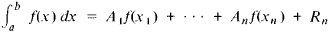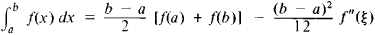The following article is from The Great Soviet Encyclopedia (1979). It might be outdated or ideologically biased.

formulas for approximating definite integrals by means of the values of the integrand at a finite number of points. The most common quadrature formulas have the formwhere x1x2, …, xn are the nodes of the quadrature formula, A1, A2 . . . ,An are its coefficients, and Rn is the remainder term. For example,where a ≤ ξ ≤ b (trapezoidal rule). Sometimes for mulas for mechanical, or numerical, quadrature are also called quadrature formulas.

### REFERENCE

Krylov, V. I. Priblizhennoe vychislenie integralov, 2nd ed. Moscow, 1967.